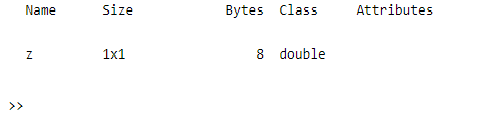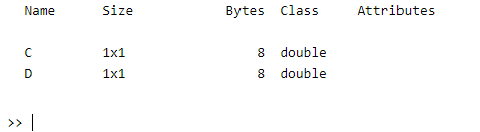# Clear variable from Memory in MATLAB

• Last Updated : 04 Jul, 2021

Clearing variables from memory, with the help of clearvars operation. The clearvars operation is used to clear the specified variables from memory or from the currently active workspace.

### Syntax:

```clearvars variables
clearvars -except keepVariables```

Parameters: This function accepts a parameter.

• variables: These are the specified variables that are going to be cleared.

Example 1

## Matlab

 `% MATLAB code for variables "x" and "y"  ``% cleared from the memory and variable "z" ``% will be as it is.``x = 5; ``% Initializing ``y = 10;``z = 15;`` ` `% Calling the clearvars operation over``% the x and y variables ``clearvars x y`` ` `% Getting the remaining variables ``whos`

Output:Example 2

## Matlab

 `% MATLAB code for all variables will be ``% cleared except "C" and "D".``A = 5;  ``% Initializing of variables``B = 10;``C = 15;``D = 20;``E = 25;`` ` `% Calling the clearvars operation to``% clear above variables except C and D``clearvars -except C D`` ` `% Getting the remaining variables ``whos`

Output:My Personal Notes arrow_drop_up